# How to Multiply Cells and Columns in Excel & Google Sheets

This tutorial demonstrates how to multiply cells and columns in Excel and Google Sheets.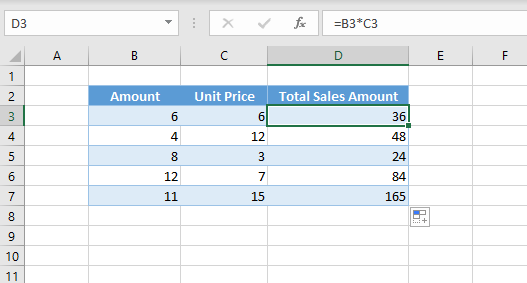Multiplication in Excel is done using the star symbol (*). You can multiply using a cell reference or by a constant.

## Multiply by a Constant

You can simply multiply two numbers and get the result in a cell. Enter the equal sign (=), the first number, the multiplication sign (*), and the second number; then press Enter. For example, the formula in cell C3 below is:

``=5*6``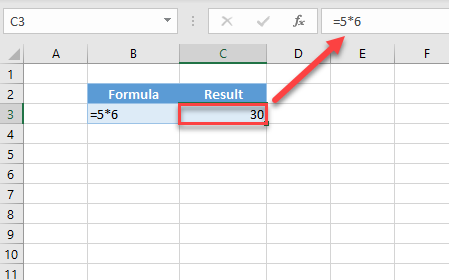The result in cell C3 is 30.

Instead of having two numbers, you can multiply a cell reference and a constant. Say you want to multiply the number in B3 by 5 and get the result in C3. To do this, enter in C3:

``=B3*5``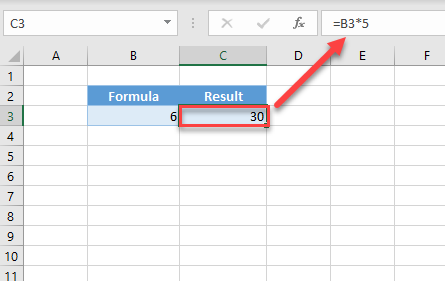The result is 30, as the value of B3 is 6. Calculating like this, the C3 value will update with a new result every time the value in B3 changes.

## Multiply Column With Cell References

As in the previous example, you can use cell references to multiply an entire column by a constant or by another cell. Say you have a table with sales data as in the picture below. In Column B, you have the amount of sold products; in Column C, you have a price per product; and in Column D, you want to calculate the total earned from the sold products. To get the result, multiply B3 by C3, so the formula in D3 is:

``=B3*C3``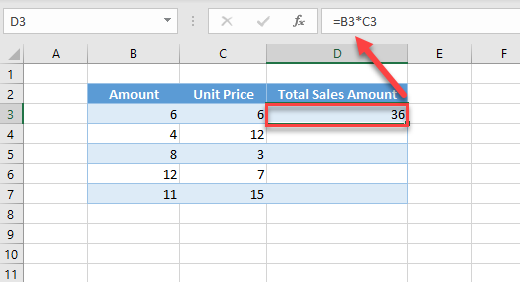The result is 36, because the amount and unit price are both 6. If you want to have all rows populated with the same formula, copy the formula down the table to Row 7.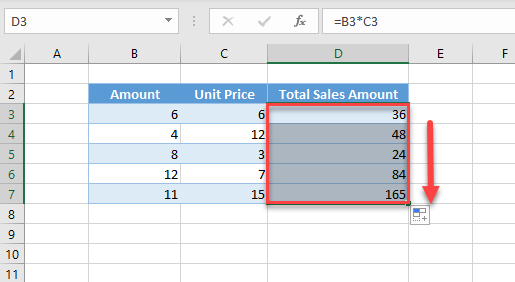As you can see, when the formula is copied, every cell in D3:D7 is now populated based on values from Columns B and C.

## Paste Special Multiply

Another way to multiply numbers is to use Paste Special. Doing this overwrites selected data with the results of your multiplication. Let’s say you have some amounts in Columns B and want to multiply them by the value in D3 (3).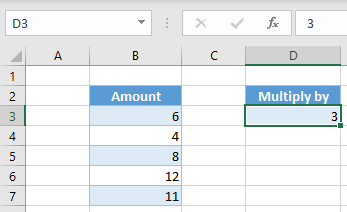1. First select cell D3, right-click on it, and click on Copy (or use the keyboard shortcut CTRL + C).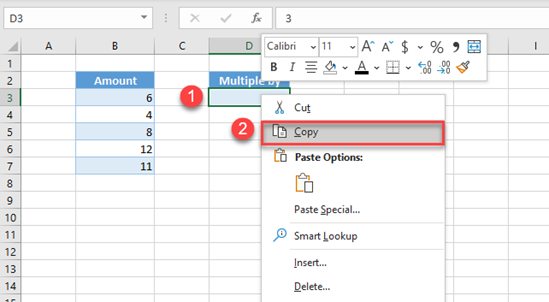1. Now, select the range you want to multiply by 5 (B3:B7), right-click on the selected area, and choose Paste Special.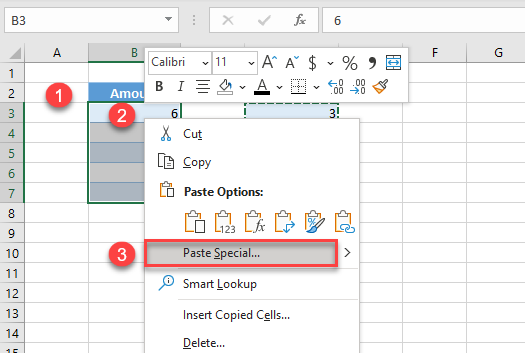1. In the Paste Special window, select Multiply, and click OK.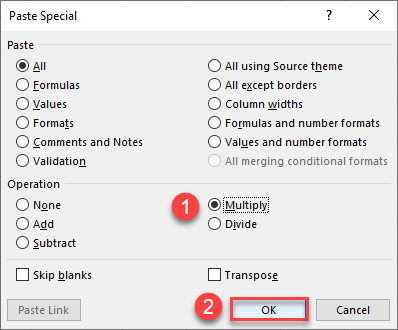As a result, all cells in the selected range are multiplied by the value from D3 (5).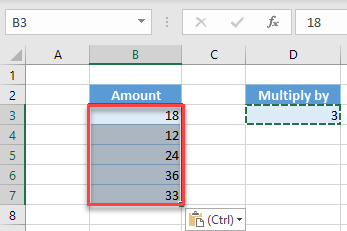Using the same methods, you can also add, subtract, or divide cells and columns in Excel.

Note: You can also multiply numbers in Excel by using the PRODUCT Function.